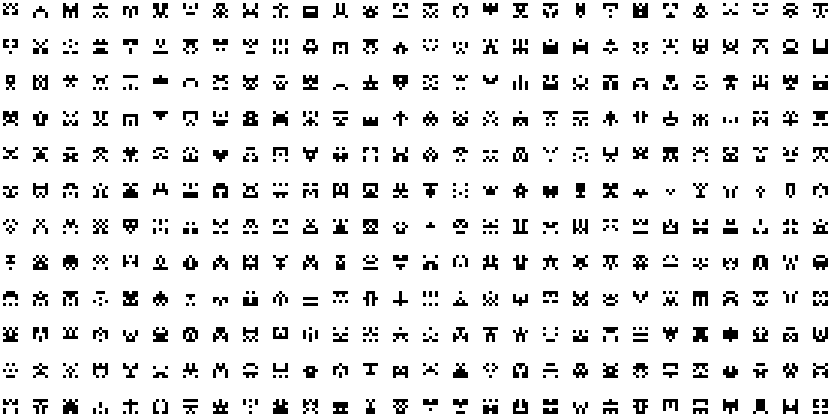Inspired by jtarbell's original fractal invaders, I took the liberty of implementing this in CHICKEN Scheme using doodle.

```(use doodle matchable (srfi 1))

(define block-width 3)
(define block-height 3)
(define block-rows 5)
(define block-cols 6)

(new-doodle width: 840
height: 420
background: solid-white)

(define (block x y row col)
(let*  ((x0 (+ x (* col block-width)))
(y0 (+ y (* row block-height)))
(x1 (+ x0 block-width))
(y1 (+ y0 block-height)))
(list x0 y0 block-width block-height)))

(define (color v)
(if (= v 0) solid-black solid-white))

(define (mirror grid)
(map (lambda (lst)
(append lst (cdr (reverse lst)))) grid))

(define (draw-blocks x y blks color-chooser)
(let loop ((bs (mirror blks))
(row 0))
(cond ((null? bs) #t)
(else
(fold (lambda (b col)
(apply filled-rectangle `(,@(block x y row col)
,(color-chooser b)))
0
(car bs))
(loop (cdr bs)

(define (random-blocks)
(list-tabulate block-rows
(lambda _
(list-tabulate (/ block-cols 2)
(lambda _ (random 2))))))

(define (random-critter! x y)
(draw-blocks x y (random-blocks) color))

(let outer ((x 3))
(if (< x doodle-width)
(begin
(let inner ((y 3))
(if (< y doodle-height)
(begin

(random-critter! x y)
(inner (+ y (* 2 block-width block-cols))))))
(outer (+ x (* 2 block-height block-rows))))))
(show!))

(define *painting* #t)

(world-inits (lambda ()

(world-changes (lambda (events dt exit)
(for-each
(lambda (e)
(match e
(('key 'pressed #\esc)
(exit #t))
(('key 'pressed #\space)
(set! *painting* (not *painting*)))
(else (void))))
events)
(when *painting*